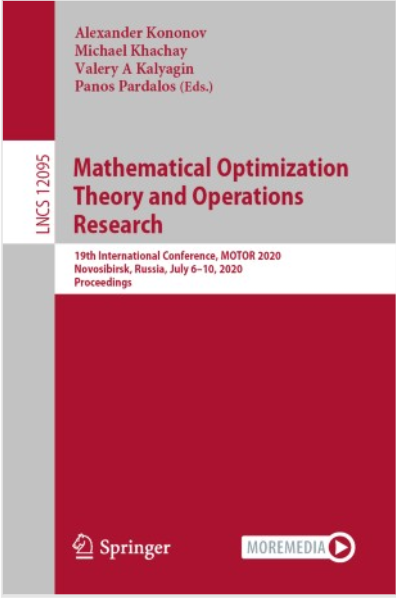• A
• A
• A
• ABC
• ABC
• ABC
• А
• А
• А
• А
• А
Regular version of the site

## On the Proximity of the Optimal Values of the Multi-dimensional Knapsack Problem with and Without the Cardinality Constraint

P. 16-22.
Чирков А. Ю., Gribanov D., Zolotykh N.

We study the proximity of the optimal value of the m-dimensional knapsack problem to the optimal value of that problem with the additional restriction that only one type of items is allowed to include in the solution. We derive exact and asymptotic formulas for the precision of such approximation, i.e. for the infinum of the ratio of the optimal value for the objective functions of the problem with the cardinality constraint and without it. In particular, we prove that the precision tends to 0.59136…/m if n→∞ and m is fixed. Also, we give the class of the worst multi-dimensional knapsack problems for which the bound is attained. Previously, similar results were known only for the case m=1.

### In bookCham: Springer, 2020.